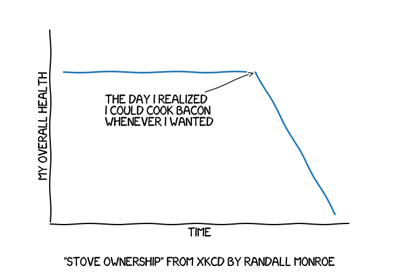# matplotlib.pyplot.xkcd¶

`matplotlib.pyplot.``xkcd`(scale=1, length=100, randomness=2)[source]

Turns on xkcd sketch-style drawing mode. This will only have effect on things drawn after this function is called.

For best results, the "Humor Sans" font should be installed: it is not included with matplotlib.

Parameters: scale : float, optional The amplitude of the wiggle perpendicular to the source line. length : float, optional The length of the wiggle along the line. randomness : float, optional The scale factor by which the length is shrunken or expanded.

Notes

This function works by a number of rcParams, so it will probably override others you have set before.

If you want the effects of this function to be temporary, it can be used as a context manager, for example:

```with plt.xkcd():
# This figure will be in XKCD-style
fig1 = plt.figure()
# ...

# This figure will be in regular style
fig2 = plt.figure()
```

## Examples using `matplotlib.pyplot.xkcd`¶XKCD